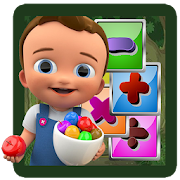# Mathzee - Learn Add, Subtract, Multiply, DivideEveryone
13
Main purpose of Mathzee app is to provide platform for children to practice of mathematical operations like Addition, Subtraction, Multiplication and Division.

Features :

* Subtraction
Learn Subtraction from this App.

* Multiplication
Learn Multiplication from this App.

* Division
Learn Division from this App.

* Maths Games
It is easy math game for children and all the people

* Math Practice
Very Good Math Practice with this app.

* Math for kids
Kids can improve their math.

* Math test
Giving answer in time for math test.

* Math question
There are mathematical questions.

* Cool math games
It is very cool game in math.

* Easy math
There is easy method to learn math.

* Math activities
With using this app anyone can doing the math activities.

* Basic math
App consider the basic mathematical operation.
Collapse

## Reviews

Review Policy
4.8
13 total
5
4
3
2
1

Updated
September 3, 2019
Size
5.6M
Installs
1,000+
Current Version
2.0.8
Requires Android
5.0 and up
Content Rating
Everyone
Permissions
Offered By
TechBeats Solution